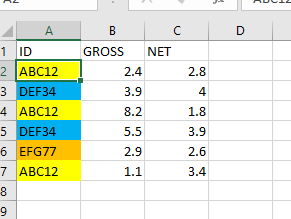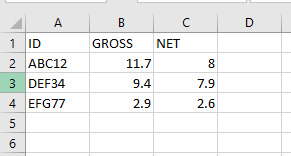# How to sum up the Amount of Dublicate Id's in Data table In Linq

Hi Team,

Here i am having the input data where the “ID” are repetitive so, here i need to sum up all the Duplicate ID Amount i.e. (GROSS and Net),

Please find the below screen shots

Input:Required Output:Input File:

Data.xlsx (9.3 KB)

You can refer to this video.

Thanks,
Sanjit

1 Like

Please try this code in the assign statement and let me know if it works,

(From row In dt.AsEnumerable
Group row By key = New With { Key .group = row.Item(“ID”) } Into grp = Group
key.group,
grp.Sum(Function(row) Convert.ToDouble(row.Item(“GROSS”).ToString)),
grp.Sum(Function(row) Convert.ToDouble(row.Item(“NET”).ToString))
}, True)
).CopyToDataTable

Regards.

1 Like

``````(From row In dtInput.AsEnumerable
Group row By sc = row("ID").ToString()
Into grp = Group
Let totalGross= grp.sum(Function (x) Convert.ToDouble(x("GROSS").ToString()))
Let totalNet= grp.sum(Function (x) Convert.ToDouble(x("NET").ToString()))
Let result = New Object() {grp(0)("ID"),totalGross, totalNet}
``````

Regards

1 Like

Hi @fernando_zuluaga
Thank you for your time. its working fine now.

Regards,